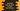# How to take one array as input from the user in JavaScript## How to take one array as input from the user in JavaScript:

In this post, we will learn how to read one array as input from the user in JavaScript. We will use a html form to take the inputs from the user and clicking on a button will show the data to the user in another HTML element.

We can either take all these inputs as comma-separated values or we can take them in different input boxes.

Let’s try both examples one by one:

### Example 1: Take all values as comma-separated:

Let’s take a look at the below example. Create one file index.html and put the below code.

``````<!DOCTYPE html>
<html lang="en">

<meta charset="UTF-8">
<meta name="viewport" content="width=device-width, initial-scale=1.0">
<title>JavaScript take inputs from user</title>

<body>
<h1>Enter all values separated by comma</h1>

<form>
<input type="text" id="arrvalues" />
<button type="button" name="button" onclick="print()">
Submit
</button>
</form>

<p id="result" />

<script type="text/javascript">

function print() {
let arrayValues = document.getElementById('arrvalues').value;
let splittedValues = arrayValues.split(',');
let resultString = "You have entered :";

for (let i = 0; i < splittedValues.length; i++) {
resultString += splittedValues[i];

if (i < splittedValues.length - 2) {
resultString += ', ';
} else if (i !== splittedValues.length - 1) {
resultString += ' and ';
}
}

document.getElementById("result").innerHTML = resultString;
}

</script>
</body>

</html>``````

Here,

• We have one form with one input and one button.
• On clicking the button, it calls the print function in the JavaScript code.

• This method is getting the values inserted in the input box.
• These values are entered as comma separated by the user. It uses split to get these values in an array.
• Then, by using a for loop, it builds the final string to show to the user. It appends all values to the variable resultString and finally, it shows that value in the paragram with id result.

It will give output as like below:

### Example 2: Take all values in different input boxes:

We can also create different or multiple input boxes and get these values from these boxes instead of using only one box. Let’s change the above example to use multiple input boxes instead of only one box:

``````<!DOCTYPE html>
<html lang="en">

<meta charset="UTF-8">
<meta name="viewport" content="width=device-width, initial-scale=1.0">
<title>JavaScript take inputs from user</title>

<body>
<h1>Enter all values separated by comma</h1>

<form>
<input type="text" name="arrvalues" />
<input type="text" name="arrvalues" />
<input type="text" name="arrvalues" />
<input type="text" name="arrvalues" />
<input type="text" name="arrvalues" />
<button type="button" name="button" onclick="print()">
Submit
</button>
</form>

<p id="result" />

<script type="text/javascript">

function print() {
let arrayValues = document.getElementsByName('arrvalues');

let resultString = "You have entered :";

for (let i = 0; i < arrayValues.length; i++) {
resultString += arrayValues[i].value;

if (i < arrayValues.length - 2) {
resultString += ', ';
} else if (i !== arrayValues.length - 1) {
resultString += ' and ';
}
}

document.getElementById("result").innerHTML = resultString;
}

</script>
</body>

</html>``````

Here,

• We have changed the ids of input components to name and we are using the same name for all inputs.
• We are using getElementsByName method to get all input components.
• We are using a for loop and reading the values of these components and similar to the example before, it builds the final resultString.

If you open this file in a browser, it will give one output as like below: